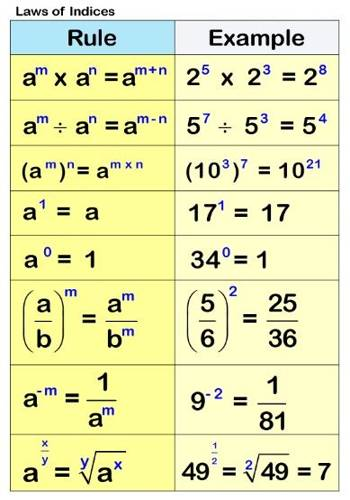Laws of Indices: Indices are a useful way of more simply expressing large numbers. They also present us with many useful properties for manipulating them using what is called the Law of Indices. Proofs for fundamental laws of indices for positive integers, a statement for fractional, zero and negative indices: simple applications.

A power, or an index, is used to write a product of numbers very compactly. The plural of index is indices. In this leaflet, we remind you of how this is done and state a number of rules, or laws, which can be used to simplify expressions involving indices.

We write the expression: 3 × 3 × 3 × 3 as 34

We read this as ‘three to the power four’.

Similarly: z × z × z = z3

We read this as ‘z to the power three’ or ‘z cubed’.

In the expression bc, the index is c and the number b is called the base. Your calculator will probably have a button to evaluate powers of numbers. It may be marked xy. Check this, and then use your calculator to verify that

74 = 2401 and 255 = 9765625Six rules of the Law of Indices

Rule 1: a0 = 1

Any number, except 0, whose index is 0 is always equal to 1, regardless of the value of the base.

Rule 2: a-m = 1/am

Rule 3: am x an = am+n

To multiply expressions with the same base, copy the base and add the indices.

Rule 4: am / an = am – n

To divide expressions with the same base, copy the base and subtract the indices.

Rule 5: (am)n = amn

To raise an expression to the nth index, copy the base and multiply the indices.

Rule 6: am/n = n√(am) = (n√a)m

Information Source: